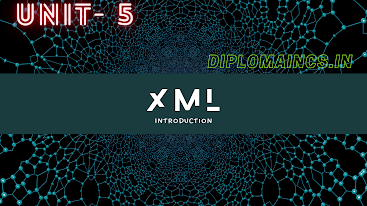## 12/21/22

### Computer programming Through C 2022 question

Sub Code: - 2000302

2022(Odd)

Time : 3Hrs

Semester  III/CSE

CPTC

Full Marks : 70

Pass marks : 28

Group A

Choose the most suitable answer from the following options: -   (1*20=20)

(i) C can be used on:

(a) Only MS-DOS operation system

(b) Only Linux operating system

(c) Only windows operating system

(d) All of the above

(ii) C programs are converted into machine language with the help of:

(a) An editor

(b) An operating system

(c) A compiler

(d) None of the above

(iii) The real constant in the C an be expresses in which of the following forms:

(a) Exponential form only

(b) Factorial form only

(c) ASCII form only

(d) Both Exponential and Factorial form

(iv) A character variable can at a time store:

(a) 1 character

(b) 8 characters

(c) 254 characters

(d) None of these

(a) An alphabet

(b) A number

(c) A special symbol other than underscore

(d) Both (b) and (c)

(vi) Which of the following is allowed in a C arithmetic instruction:

(a) []

(b) {}

(c) ()

(d) None of these

(vii) The break statement is used to exit from:

(a) An if statement

(b) A for loop

(c) A program

(d) The main () function

(viii) A do-while loop is useful when we want that the statement within the loop must be executed:

(a) Only once

(b) At least once

(c) More than once

(d) None of the above

(ix) Which of the following statement is used to take the contrail to the beginning of the loop.

(a) Exit

(b) Break

(c) Continue

(d) None of the above

(x) Which of the following is not a preprocessor directive:

(a) #if

(b) #elseif

(c) #undef

(d) #pragma

(xi) All macro substitution in a program are done:

(a) Before compilation of the program

(b) After- compilation

(c) During compilation

(d) None of the above

(xii) What will happen if you assign a value to an element of an array whose subscript exceeds the size of the array?

(a) The element will be set to 0 (zero)

(b) Error message from the compiler

(c) Other data may be overwritten

(d) None of the above

(xiii) When you pass an array as an argument to a function, what actually gets passed?

(b) Values of the element of the array

(c) Address of the first element of the array

(d) Number of elements of the array

(xiv) If you don’t initialize a static array, what would be the element set to?

(a) 0 (zero)

(b) An undetermined value

(c) A floating point numbers

(d) The character constant ‘/0’

(xv) If int S is a one-dimensional array of integers, which of the following refers to the third element in the array?

(a) *(S+2)

(b) *(S+3)

(c)  S+3

(d) S+2

(xvi) If an integer is to be entered through the keyboard, which function would you use?

(a) scanf()

(b) gets()

(c)  getche()

(d) getchar()

(xvii) Following one keyboard is used for function not returning any value.

(a)  void

(b) int

(c)  auto

(d) None of the above

(xviii) Recursion is a process in which a function calls:

(a)  Another function

(b) main () function

(c)  itself

(d) None of these

(xix) The number variable of a structure is accessed by using:

(a)  arrow (->) operator

(b) dot(.) operator

(c)  asterisk (*) operator

(d) ampersand (&) operator

(xx) The union holds

(a)  One object at a time

(b) Multiple objects

(c) Both (a) and (b)

(d) None of the above

Group:-"B"

Answer all Five Questions: -                         (5*4=20)

2.  What is structure in C? How is a structure declared?

OR

What is union in C? How is data stored using union?

3.  Explain lifetime and visibility of a variable.

OR

List and explain the four scopes variable in brief.

4. How do functions help to reduce the program size?

OR

Differentiate between library and user defined functions.

5.  What are actual and formal arguments?

OR

Explain the type of recursion.

6. What is the main () function in C? Why is it necessary in each program?

OR

Mention differences between character array and integer array.

Group:- "C"

Answer all Five Questions: -                         (5*6=30)

7.  Write a program to demonstrate the use of three- dimensional array.

OR

Write a program to perform addition and subtraction of two matrices whose orders up to 10 *10.

8. Compute the factorial of the give number using the do-while loop.

OR

Write a program to enter a number through keyboard and find sum of its digits.

9.  Write a program to calculate bill of internet browsing the condition are given below.

a.    1 hours – 20 Rs.

b.    ½ hours- 10 Rs

c.     Unlimited hours in a day -90 Rs. Owner should enter number of hours spent by customer.

OR

Explain different types of operators supported by C.

10.  Elaborate different sections of a C program.

OR

Describe the various function used for memory allocation.

11.  If the ages of Ram, Shyam and Ajay are input through the keyboard, write a program to determine the youngest of the three.

OR

Write a program to calculate the perimeter and area of rectangle & circle.

### To access previous year question of the year 2017 to 2021